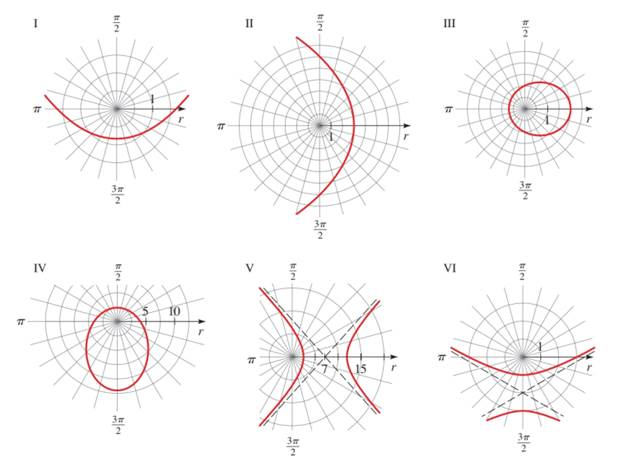Chapter 12.6, Problem 14E### Algebra and Trigonometry (MindTap ...

4th Edition
James Stewart + 2 others
ISBN: 9781305071742

#### Solutions

Chapter
Section### Algebra and Trigonometry (MindTap ...

4th Edition
James Stewart + 2 others
ISBN: 9781305071742
Textbook Problem

# 11-26 Graphs of polar equation of conics Match the following equation with the graphs labeled I-VI. Give reason for your answer.14. r = 5 3 − 3 sin θTo determine

Nature of graph the graphs labeled I-VI for the conic equation r=533sinθ and reason for the answer.

Explanation

Given:

The given conic equation,

r=533sinθ

θ is angle

Approach:

Compare the given equation with the standard equation. r=ed1esinθ…(1)

Then,

e<1,it is an ellipsee=1it is a parabolae>1it is a hyperbola

Write the equation of directrix

### Still sussing out bartleby?

Check out a sample textbook solution.

See a sample solution

#### The Solution to Your Study Problems

Bartleby provides explanations to thousands of textbook problems written by our experts, many with advanced degrees!

Get Started